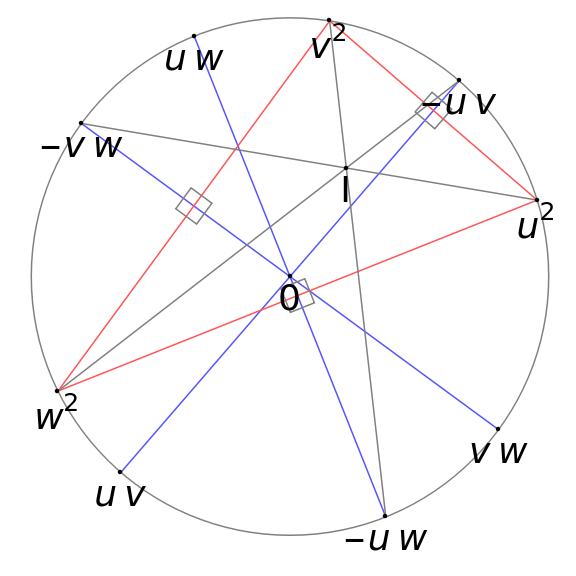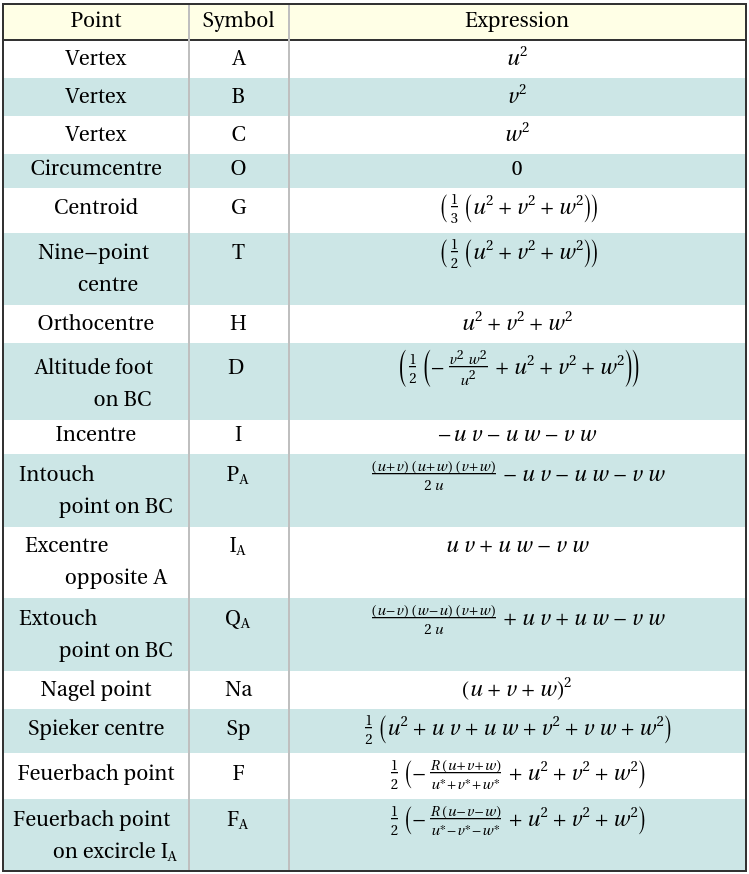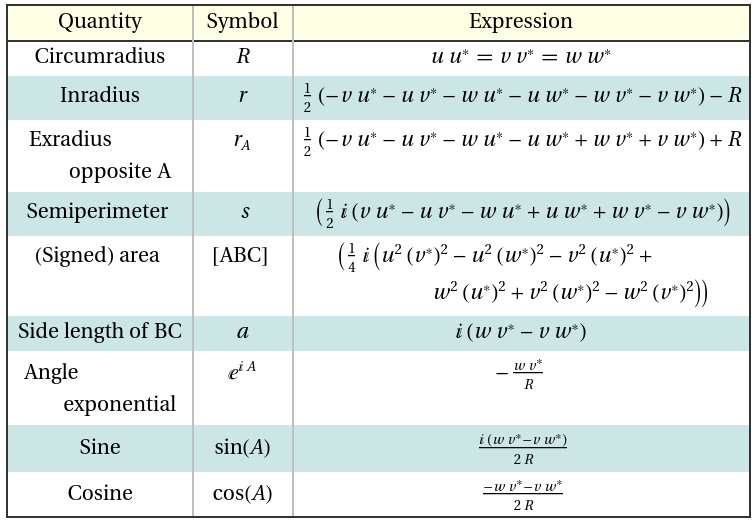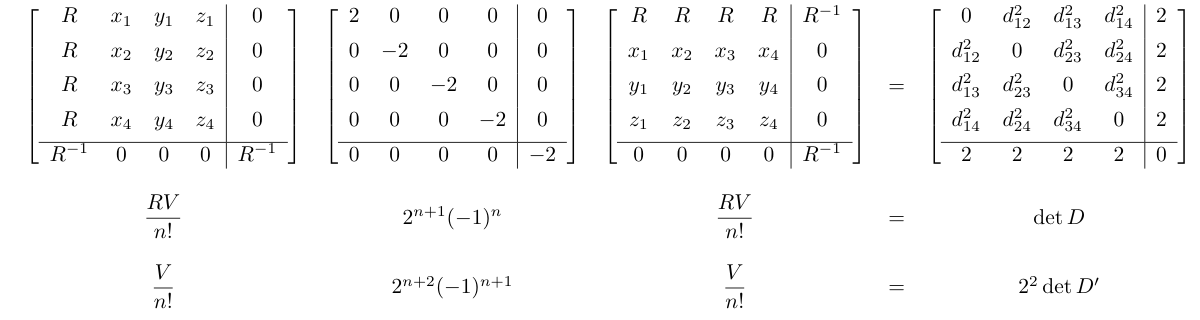# Let the circumcentre be your origin

Suppose we have two vectors, u and v, in a Euclidean vector space. If we wanted to somehow quantify the proximity of these two vectors, there are two particularly appealing choices:

• the squared distance, |uv|²;
• the inner product, u.v;

Indeed, the only isotropic (invariant under rotations/reflections of the ambient space) functions of u, v that can be expressed as polynomials of degree ≤ 2 in the entries of the vectors are precisely the linear combinations of |uv|², u.v, and 1. Conversely, if we know both the squared distance and the inner product, we can completely recover the pair of vectors up to rotations/reflections of the ambient space.

Both (squared) distances and inner products are very useful in practice, and it seems unsatisfying to have to choose between them. Fortunately, there’s a common situation in which you don’t need to do so: that’s when all of your points lie on an origin-centred sphere! In particular, if u and v both lie on an origin-centred sphere of radius R, we have:

|uv|² = 2(R² − u.v)

and conversely:

u.v = R² − ½|uv

so we can compute either of these quantities given the other one.

There are many applications in machine learning in which you want to compute the matrix of distances between a set of points in some latent space. If you’ve constrained the latent embedding to force everything onto the unit sphere, then this can be done very efficiently: you just compute the pairwise dot-products by a single multiplication of a matrix by its transpose, and then apply a simple elementwise transformation to convert these inner products into distances.

Often we don’t have the liberty to impose constraints on where our points lie, so having them be on an origin-centred sphere cannot be guaranteed. There is, however, one important exception:

### Simplices

A non-degenerate simplex (i.e. a triangle, tetrahedron, or higher-dimensional analogue thereof) has a unique circumcentre, a point equidistant from all of the vertices. If you’re trying to reason about the geometry of a simplex, then you can firstly translate it so that this circumcentre coincides with the origin.

A helpful heuristic in solving Euclidean geometry problems concerned with a triangle is to ‘always draw the circumcircle’, and the approach of setting the circumcentre to be the origin is a natural extension of this. In Mathematical Olympiad Dark Arts (which I’m in the process of revising ready for publication as both books and online courses), this is the starting point for an algebraically convenient way to parameterise a triangle by complex numbers where the vertices are u², v², and w²:By judiciously choosing the signs of u,v,w to ensure the angle bisectors meet the circle again at −vw, −uw, and −uv (this can be guaranteed), many of the most important triangle centres have positions given by homogeneous quadratic polynomials (or, failing that, rational functions) in u, v, w:Similarly, important scalars associated with the triangle (such as the circumradius, inradius, semiperimeter, side-lengths, and so forth) are expressible as homogeneous polynomials in the parameters and their complex conjugates:There’s actually a ‘strong type system’ lurking in here: we say that the parameters u, v, w have type (0, 1) and their conjugates have type (1, 0). Ordinary ‘dimensionless’ complex numbers (such as pi, i, and 2) have type (0, 0). Then we have the rules that if you multiply quantities, their types add elementwise, and you are only allowed to add/subtract quantities of the same type, and apply transcendental functions (such as exp) to ‘dimensionless’ quantities. In this type system, the following hold:

• all points in the plane of the triangle have type (0, 2);
• all lengths have type (1, 1);
• all areas have type (2, 2);

and this type system helps catch any symbolic errors you might make when manually manipulating these expressions.

### Cayley-Menger determinants

These are formulae for the volumes of simplices using only their squared side-lengths. We’ve looked at them before, where we used elementary row/column operations to manipulate determinants in order to prove their correctness. But with the trick of letting the circumcentre be the origin, we can much more succinctly prove the Cayley-Menger formula and a variant thereof.

In particular, here is the example for a tetrahedron (n = 3); it should be straightforward to see how it generalises:Firstly, convince yourself that the matrix equation (first row) is true. It relies on what we’ve discussed about the relationship between dot-products and squared distances.

Observe the middle matrix, which is diagonal and has a signature of (1, n+1). You can think of this product as computing the (doubled) pairwise Lorentzian inner products of the rows of the leftmost matrix. The ‘time’ coordinate (first entry in each row) of the leftmost matrix is visibly equal to the norm of the ‘space’ coordinates (remaining entries), which is why each row has Lorentzian norm of zero (and therefore the diagonal of the product of the matrices is 0).

The two scalar equations below the matrix equation are, respectively:

• the determinants of the upper-left submatrices of dimension n+1 (i.e. the matrices after the bottom row and rightmost column are removed);
• the determinants of the full matrices of dimension n+2;

and the equations hold because determinants are multiplicative.

In the case of triangles, the first scalar equation simplifies to the theorem that the area of a triangle is abc/4R, where a,b,c are the side-lengths and R is the circumradius. The second scalar equation simplifies to Heron’s formula for the area of a triangle.

This entry was posted in Uncategorized. Bookmark the permalink.